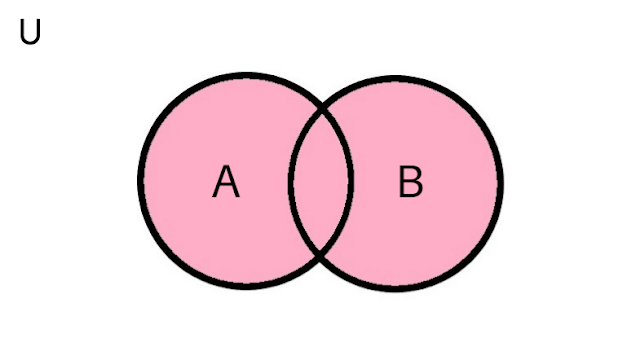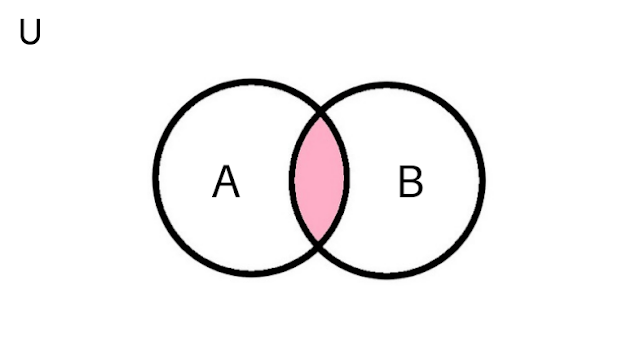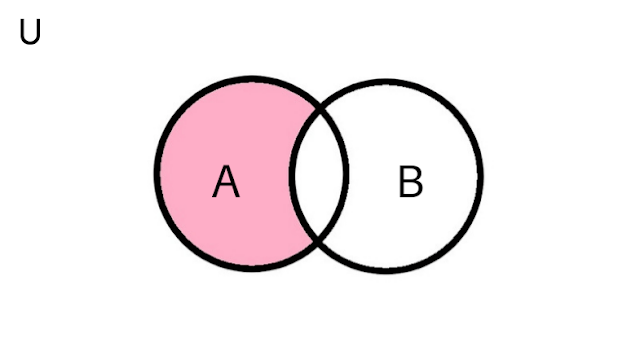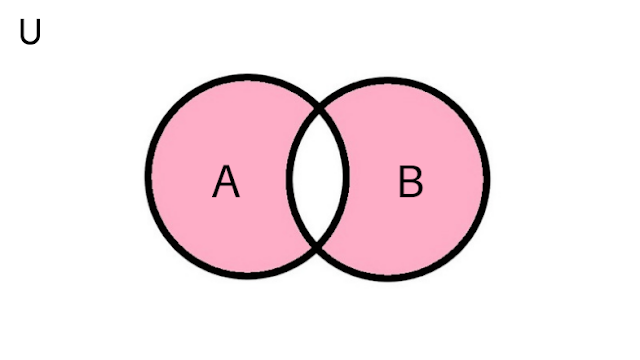# Python Sets

Here, we have discussed about python set. Here, we have learnt what is python set, how to create python set, modifying/adding elements to set,...

In this article, we will learn about what is python set, how to create python set, modifying & adding elements to set, deleting elements from a set, python set operations, python set methods and python set built-in functions.

## What are Python sets?

In python, set is a collection of elements in an unordered manner. The set contains those data types which are iterable and immutable. And each elements of set are unique which means that there are no duplicates of these elements. Python sets contains immutable elements but the are sets are mutable itself which means we can make changes in a set after its creation.

We can't access the elements of a set by using the indexing method because the elements of set are in unordered sequence. With the help of sets, we can perform many other mathematical operations like symmetric difference, union, intersection, etc.

## Creating a Python Set

We can create a set by writing the iterable and immutable elements within the Curly brackets {}, all these elements are separated by commas (,). Python also provides the set() built-in function with which we can create a set. A set may contain various number of items and they can be of any type like float, integers, tuple, strings, etc. but the only requirement is that they can't be mutable.

`# There are different types of sets in Python programming language# Python set of integersset1 = {14, 7, 21}print(set1)# Python set of mixed datatypesset2 = {7.6, "I Love Python", "Answersjet", (14, 7, 21)}print(set2)`

Output

`{21, 14, 7}{'Answersjet', (14, 7, 21), 'I Love Python', 7.6}`

You can also try the following illustration as well:

`# In python, set cannot have duplicatesset1 = {4, 5, 6, 7, 6, 5}print(set1)# we can make set from a listset2 = set([4, 5, 6, 5])print(set2)# In Python, set cannot have mutable items# below [14, 9] is a mutable list which will cause an error.set3 = {4, 5, [14, 9]}print(set3)`

Output

```{4, 5, 6, 7}
{4, 5, 6}
Traceback (most recent call last):  File "<string>", line 9, in <module>
TypeError: unhashable type: 'list'```

Python sets are mutable but the elements of sets are immutable and in unordered manner, so we can't able to access or change the elements of a set by either slicing or indexing method. But python allows us to add elements in a set by the following ways;

By using add() method we can add only a single element to the set.

`# A Python program to demonstrate adding elements in a set# initialize friends friends = {'Deepak', 'Ritik', 'Saatvik'}print(friends)# add an elementfriends.add('Rahul')print(friends)`

Output

`{'Saatvik', 'Deepak', 'Ritik'}{'Rahul', 'Saatvik', 'Deepak', 'Ritik'}`

### Using update() method

With the help of update() method we can add multiple elements to a set. The update() method supports other data types as argument such as list, string, tuple or other sets.

`# Python program to demonstrate to updating elements in setsfriends = {"Deepak", "Ritik", "Saatvik"}print(friends)# add multiple elementsfriends.update(["Rahul", "Suraj", "Rohit"])print(friends)# add list and setfriends.update(["Rahul", "Suraj"], {"Rohit", "Anuj", "Rishav"})print(friends)`

Output

```{'Saatvik', 'Deepak', 'Ritik'}
{'Suraj', 'Rohit', 'Rahul', 'Ritik', 'Saatvik', 'Deepak'}
{'Suraj', 'Rohit', 'Rishav', 'Rahul', 'Anuj', 'Ritik', 'Saatvik', 'Deepak'}```

## Deleting elements from a Set

In python, we can delete or remove the elements of a set by the following methods;

### Using discart() method

We can remove the items of a set by using the discard() function. If we are using discard() function on a elements and that element is not present in the set then the set will remain unchanged.

`# A Python program to demonstrate discard elements from a set# initialize set1set1 = {11, 12, 13, 14, 15}print(set1)# discard an elementset1.discard(14)print(set1)# discard an element that not present in set1set1.discard(16)print(set1)`

Output

```{11, 12, 13, 14, 15}
{11, 12, 13, 15}
{11, 12, 13, 15}```

### Using remove() method

In python, we also have the remove() built-in function with which we can remove the elements of a set. But unlike discard() method, if the element is not present in the set then it will result in error.

`# A Python program to demonstrate removing elements from a set# initialize set1set1 = {11, 12, 13, 14, 15}print(set1)# remove an element from set1set1.remove(14)print(set1)# remove an element that are not present in set1# It will give an errorset1.remove(16)print(set1)`

Output

```{11, 12, 13, 14, 15}
{11, 12, 13, 15}
Traceback (most recent call last):
File "<string>", line 9, in <module>
KeyError: 16```

## Python Set Operations

Python sets are also used to perform many mathematical operations which are explained as follows;

### Set UnionSet Union in Python

In python, the union of two sets is calculated with the help of (|) operator. And we can also use the union() method to find out the union of two sets. All the elements of two sets are included in the union of those two sets.

`# A Python program to demonstrate set union with the help of | operator# initialize x1 and x2x1 = {11, 12, 13, 14, 15}x2 = {14, 15, 16, 17, 18}# use | operatorprint(x1 | x2)`

Output

`{11, 12, 13, 14, 15, 16, 17, 18}`

You can also try this example on shell:

`# A Python program to demonstrate set union by using union function# use union function on x1>>> x1.union(x2){11, 12, 13, 14, 15, 16, 17, 18}# use union function on x2>>> x2.union(x1){11, 12, 13, 14, 15, 16, 17, 18}`

### Set IntersectionSet Intersection in Python

In python, the intersection of two sets is calculated with the help of (&) operator. And we can also use the intersection() method to find out the intersection of given two sets. The common elements of both sets are included in the intersection of those two sets.

`# A Python program to demonstrate set intersection with the help of & operator# initialize x and yx = {11, 12, 13, 14, 15}y = {14, 15, 16, 17, 18}# using & operatorprint(x & y)`

Output

`{14, 15}`

You can also try this example on shell:

`# A Python program to demonstrate set intersection by using intersection function# use intersection function on x>>> x.intersection(y){14, 15}# use intersection function on y>>> y.intersection(x){14 , 15}`

### Set DifferenceSet Difference in Python

With the help of (-) operator, we calculate the difference of two sets. And we can also use the difference() method to find out the difference of two sets. In the difference of two sets those elements are included which are present in the first set but not presented in the second set

`# A Python program to demonstrate set difference with the help of - operator# Difference of two sets# initialize x and yx = {11, 12, 13, 14, 15}y = {14, 15, 16, 17, 18}# using - operator on xprint(x - y)`

Output

`{11, 12, 13}`

You can also try this example on shell:

`# A Python program to demonstrate set difference with the help of difference function# using difference function on x>>> x.difference(y){11, 12, 13}# using - operator on y>>> y - x{18, 16, 17}# using difference function on y>>> y.difference(x){18, 16, 17}`

### Set Symmetric DifferenceSet Symmetric Difference in Python

In python, the symmetric difference of two sets are calculated with the help of [^] operator and also by using [symmetric_difference()] method. In Symmetric Difference, the elements of both sets are included except those elements which lies in the intersection.

`# A Python program to demonstrate set Symmetric difference with the help of  ^ operator# Symmetric difference of two sets# initialize x and yx = {11, 12, 13, 14, 15}y = {14, 15, 16, 17, 18}# using ^ operatorprint(x ^ y)`

Output

`{16, 17, 18, 11, 12, 13}`

You can also try this example on shell:

`# A Python program to demonstrate set  Symmetric difference with the help of symmetric difference function# using symmetric_difference function on x>>> x.symmetric_difference(y){16, 17, 18, 11, 12, 13}# using symmetric_difference function on y>>> y.symmetric_difference(x){16, 17, 18, 11, 12, 13}`

## Python Set Methods

The following is the list of all python set methods with description;

Methods Description
update() To update a set with another set.
discard() To remove specified element from the set.
frozenset() To return immutable frozenset objects.
difference()Used to return a set with the difference of two sets.
difference_update() To insert the difference of two sets in the existing caller set.
symmetric_difference() Used to return a set with the symmetric difference of two sets.
symmetric_difference_update()To insert the symmetric difference of two sets in the existing caller set.
intersection()Used to return a set that contains the intersection of two sets.
intersection_update() To insert the interstate of two sets in the existing caller set.
pop() To remove any random element from the set.
remove()To remove an element from the set.
union()The set containing union of sets is returned.
clear() To remove all elements of a set.
copy() To return the copy of the set.
isdisjoint()To check whether two sets have intersection between them or not.
issubset() To check whether a set is subset of another or not.
issuperset() To check whether a set is superset of another set or not.

## Built-in functions in Set

The list of all built-in function that are used in set is given as follows;

S.No Function Description
1. any() If any element of the set is true then it returns true and if the set is empty then it returns false.
2. all() If the list is empty or all the elements of set are true then it returns true.
3. sum() To sum up the numbers of elements in the set.
4. min()To return the smallest or minimum element of a set.
5. max()To return the highest or maximum element of a set.
6. enumerate() To return the enumerate object of a set.
7. sorted() To return a sorted version of elements of a set.
8. len()To set the length or size of the list.

## Python Frozensets

Python Frozenset is a new class which contains sets that are immutable. These have the characteristics of a set but we can't change the elements of Frozenset after being assigned. In simple terms we can say that, we can modify the elements of a set but the elements of Frozensets can't be modified. One can create Frozensets by using frozenset() function. We can also use frozenset as keys to a dictionary because these are hashable.

`# Python Frozensets example# initialize x and yx = frozenset([11, 12, 13, 14])y = frozenset([13, 14, 15, 16])`

Try these examples on shell :

`>>> x.isdisjoint(y)False>>> x.difference(y)frozenset({11, 12})>>> x | yfrozenset({11, 12, 13, 14, 15, 16})>>> x.add(13)...AttributeError: 'frozenset' object has no attribute 'add'`

## Conclusion

Above we have discussed about python set. Here, we have learnt what is python set, how to create python set, modifying/adding elements to set, deleting elements from a set, python set methods and python set built-in functions. In python, set is a collection of elements in an unordered manner. The set contains those data types which are iterable and immutable.